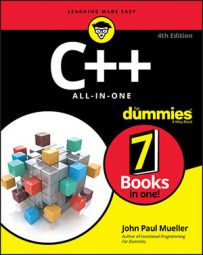##### C++ All-in-One For DummiesThe name of the array is a pointer to the array itself. The array is a sequence of variables stored in memory. The array name points to the first item.

This is an interesting question about pointers: Can you have a function header, such as the following line, and just use sizeof to determine how many elements are in the array? If so, this function wouldn’t need to have the caller specify the size of the array.

`int AddUp(int Numbers[]) {`

Consider this function found in the Array01 example and a main() that calls it:

```void ProcessArray(int Numbers[]) {
cout << "Inside function: Size in bytes is "
<< sizeof(Numbers) << endl;
}
int main(int argc, char *argv[])
{
int MyNumbers[] = {1,2,3,4,5,6,7,8,9,10};
cout << "Outside function: Size in bytes is ";
cout << sizeof(MyNumbers) << endl;
ProcessArray(MyNumbers);
return 0;
}```

When you run this application, here’s what you see:

```Outside function: Size in bytes is 40
Inside function: Size in bytes is 4```

Outside the function, the code knows that the size of the array is 40 bytes. But why does the code think that the size is 4 after it is inside the array? The reason is that even though it appears that you’re passing an array, you’re really passing a pointer to an array. The size of the pointer is just 4, and so that’s what the final cout line prints.

Declaring arrays has a slight idiosyncrasy. When you declare an array by giving a definite number of elements, such as

`int MyNumbers;`

the compiler knows that you have an array, and the sizeof operator gives you the size of the entire array. The array name, then, is both a pointer and an array! But if you declare a function header without an array size, such as

`void ProcessArray(int Numbers[]) {`

the compiler treats this as simply a pointer and nothing more. This last line is, in fact, equivalent to the following line:

`void ProcessArray(int *Numbers) {`

Thus, inside the functions that either line declares, the following two lines of code are equivalent:

```Numbers = 10;
*(Numbers + 3) = 10;```

This equivalence means that if you use an extern declaration on an array, such as

`extern int MyNumbers[];`

and then take the size of this array, the compiler will get confused. Here’s an example: If you have two files, numbers.cpp and main.cpp, where numbers.cpp declares an array and main.cpp externally declares it (as shown in the Array02 example), you will get a compiler error if you call sizeof:

```#include <iostream>
using namespace std;
extern int MyNumbers[];
int main(int argc, char *argv[])
{
cout << sizeof(MyNumbers) << endl;
return 0;
}```

In Code::Blocks, the gcc compiler gives us this error:

`error: invalid application of 'sizeof' to incomplete type 'int []'`

The solution is to put the size of the array inside brackets. Just make sure that the size is the same as in the other source code file! You can fake out the compiler by changing the number, and you won’t get an error. But that’s bad programming style and just asking for errors.

Although an array is simply a sequence of variables all adjacent to each other in memory, the name of an array is really just a pointer to the first element in the array. You can use the name as a pointer. However, do that only when you really need to work with a pointer. After all, you really have no reason to write code that is cryptic, such as *(Numbers + 3) = 10;.

The converse is also true. Look at this function:

```void ProcessArray(int *Numbers) {
cout << Numbers << endl;
}```

This function takes a pointer as a parameter, yet you access it as an array. Again, don't write code like this; instead, you should understand why code like this works. That way, you gain a deeper knowledge of arrays and how they live inside the computer, and this knowledge, in turn, can help you write code that works properly.

Even though, the array name is just a pointer, the name of an array of integers isn’t the exact same thing as a pointer to an integer. Check out these lines of code (found in the Array03 example):

```int LotsONumbers;
int x;
LotsONumbers = &x;```

Point the LotsONumberspointer to something different: something declared as an integer. The compiler doesn’t let you do this; you get an error. That wouldn’t be the case if LotsONumbers were declared as int *LotsONumbers; then this code would work. But as written, this code gives you a compiler error. And believe it or not, here’s the compiler error you get in Code::Blocks:

`error: incompatible types in assignment of 'int*' to 'int '`

This error implies the compiler does see a definite distinction between the two types, int* and int[]. Nevertheless, the array name is indeed a pointer, and you can use it as one; you just can’t do everything with it that you can with a normal pointer, such as reassign it.

• Keep your code consistent. If you declare, for example, a pointer to an integer, do not treat it as an array.

• Keep your code clear and understandable. If you pass pointers, it’s okay to take the address of the first element, as in &(MyNumbers) if this makes the code clearer — though it’s equivalent to just MyNumbers.

• When you declare an array, always try to put a number inside the brackets, unless you are writing a function that takes an array.

• When you use the extern keyword to declare an array, go ahead and also put the array size inside brackets. But be consistent! Don’t use one number one time and a different number another time. The easiest way to be consistent is to use a constant, such as const int ArraySize = 10; in a common header file and then use that in your array declaration: int MyArray[ArraySize];.